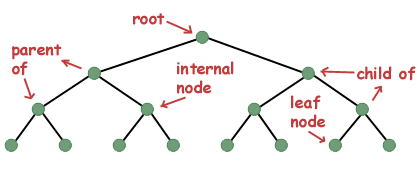# Difference between Tree and Graph Data Structure

In this tutorial you will learn about the difference between tree and graph.

Both trees and graphs are two well known mostly used data structures in algorithms.

Tree Data Structure

In Computer science, a tree is a widely used Abstract Data Structure (ADT). It can be defined recursively as a collection of nodes, where each node contains a value, starting with root node and list of references to other nodes (children), with the constraint, that no reference from it, is called leaf node.

Graph Data Structure

In Computer science, graph also an abstract data structure (ADT), that is mean to implement undirected graph and directed graph concepts of mathematics especially the field of graph theory. Graph is a mathematical representation of a set of objects and relationships or links between objects. We represent objects as nodes or vertices in graph and relations between vertices as edges or arcs. So, we can define that graph is set of vertices V and set of edges E. These edges may be directed or undirected.

Now let’s see what are the differences between graph and tree in tabular form.

## Difference between Tree and Graph

 Trees Graphs 1. A tree is a special kind of graph that there are never multiple paths exist. There is always one way to get from A to B. 1. A graph is a system that has multiple ways to get from any point A to any other point B. 2. Tree must be connected. 2. Graph may not be connected. 3. Since it connected we can reach from one particular node to all other nodes. This kind of searching is called traversal. 3. Traversal always not applicable on graphs. Because graphs may not be connected. 4. Tree contains no loops, no circuits. 4. Graph may contain self-loops, loops. 5. There must be a root node in tree. 5. There is no such kind of root node in graphs 6. We do traversal on trees. That mean from a point we go to each and every node of tree. 6. We do searching on graphs. That means starting from any node try to find a particular node which we need. 7. pre-order, in-order, post-order are some kind of traversals in trees. 7. Breath first search, Depth first search, are some kind of searching algorithms in graphs. 8. Trees are directed acyclic graphs. 8. Graphs are cyclic or acyclic. 9. Tree is a hierarchical model structure. 9. Graph is network model. 10. All trees are graphs. 10. But all graphs are not trees. 11. Based on different properties trees can be classified as Binary tree, Binary search tree, AVL trees, Heaps. 11. We differ the graphs like directed graphs and undirected graphs. 12. If tree have “n” vertices then it must have exactly “n-1” edges only. 12. In graphs number of edges doesn’t depend on the number of vertices. 13. Main use of trees is for sorting and traversing. 13. Main use of graphs is coloring and job scheduling. 14. Less in complexity compared to graphs. 14. High complexity than trees due to loops.

### Example

Tree:Graph:Comment below if you have queries or found any information incorrect in above tutorial for difference between tree and graph data structure.

### 4 thoughts on “Difference between Tree and Graph Data Structure”

1.Vasile Mironeasa

Hi Neeraj !
I want to colaborate with you to discover an algorithm for change the control of the world . I like your passion for programming ! I think that you have the vision of algorithm programming wich I do have not . If you want to help me for , please write me !
My respect to you Neeraj !
Vasile Mironeasa

2.THANK YOU SO MUCH I LEARNED MANY THINGS FROM THIS WEBSITE

3.Thanks a lot

4.Thanks a lot.But How Can I meet Sophie Turner(Sansa Stark–>GOT).Can anybody tell me the way.